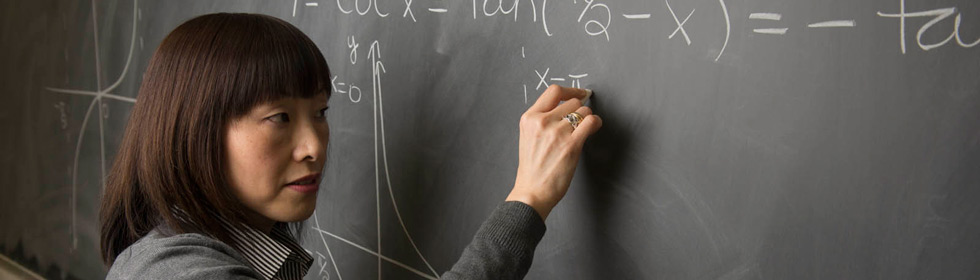Department of Mathematics & Statistics#### Minors

The Department offers a minor in Mathematics and a minor in Statistics; in fact, students can earn BOTH a mathematics AND a statistics minor!

A minor in Mathematics consists of MATH 150 and 152 (Calculus I and II), and nine hours of Mathematics (Statistics or Operations Research) courses at the 200 level or above, of which six hours must be at the 300 level or above and at least three of these six hours must be from Mathematics.

A minor in Statistics consists of MATH 150 and 152 and nine additional hours of Statistics courses at the 300 level or above.  For instance, this might include Stat 380 (statistics with applications for engineers/scientists), Stat 482 (regression), and Stat 481 (Design of Experiments with applications).

For both minors, at least six hours of courses at the 300 level or above must be taken at SIUE. Students must receive a C or better in all courses which count toward the minor.

Students majoring in Mathematical Studies may not minor in Mathematics, Statistics, or Mathematics Education.